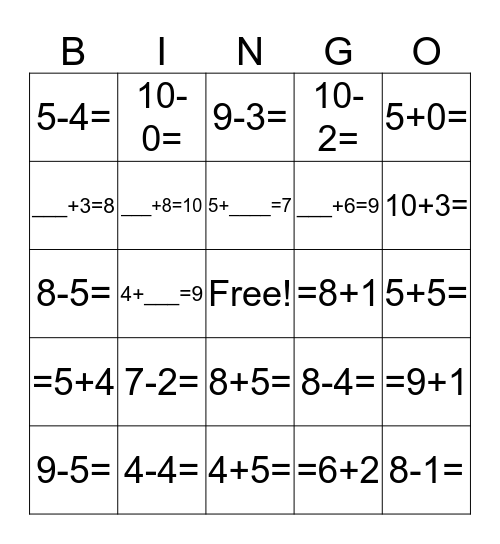# Math BingoThis bingo card has a free space and 44 words: 7+3=, 8-1=, 1+0=, 9-5=, 9+5=, =6+2, 9-3=, 7+3=, 5+0=, 4-4=, 10-2=, =8+1, 8+5=, 7-2=, 2+__=7, 4+5=, 5+4=, 8-5=, 7+0=, 10+3=, 10-0=, 3+6=, =2+10, 5-4=, 3+3=, 5+5=, =6+9, 7-__=0, 8-4=, =5+4, 9-__=0, =9+1, 10=___+5, 7=5+___, 4+___=9, 2+___=6, 3+___=9, 4+____=10, 5+____=7, ___+3=8, ___+6=9, ___+3=6, ___+8=10 and ___+ 6=10.

⚠ This card has duplicate items: 7+3= (2)

## Play Online

Share this URL with your players:For more control of your online game, create a clone of this card first.

## Probabilities

With players vying for a you'll have to call about __ items before someone wins. There's a __% chance that a lucky player would win after calling __ items.

Tip: If you want your game to last longer (on average), add more unique words/images to it.